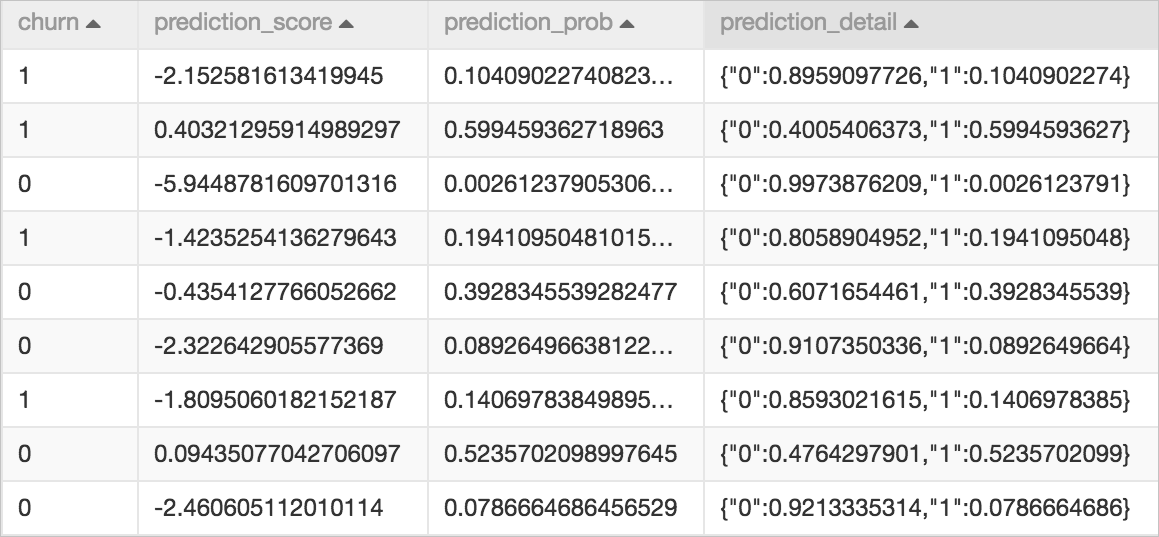The Scorecard Prediction component uses the model that is generated by the Scorecard Training component to predict scores.

## Configure the component

You can configure the component by using one of the following methods:
• Use the Machine Learning Platform for AI console
Tab Parameter Description
Fields Setting Feature Column The feature columns that are used in prediction. By default, all feature columns are selected.
Columns Reserved in Result Table The columns that are appended to the prediction result table without processing, such as the ID and objective columns.
Output Variable Score Specifies whether to generate a score for each feature variable. The total predicted score is the score of intercept options plus the score of each variable.
Tuning Number of Cores The number of CPU cores that are required. By default, the system determines the value.
Memory Size per Core The memory size of each CPU core. By default, the system determines the value.
• Use commands
``````pai -name=lm_predict
-project=algo_public
-DinputFeatureTableName=input_data_table
-DinputModelTableName=input_model_table
-DmetaColNames=sample_key,label
-DfeatureColNames=fea1,fea2
-DoutputTableName=output_score_table``````
Parameter Description Required Default value
inputFeatureTableName The name of the input feature table. Yes N/A
inputFeatureTablePartitions The partitions that are selected from the input feature table. No Full table
inputModelTableName The name of the input model table. Yes N/A
featureColNames The feature columns that are selected from the input table. No All columns
metaColNames The columns that do not need to be converted. These columns in the output are the same as those in the input. You can specify labels and sample_id in the columns. No N/A
outputFeatureScore Specifies whether to generate the scores of variables in the prediction results. Valid values:
• true: indicates that the system generates the scores of variables in the prediction results.
• false: indicates that the system does not generate the scores of variables in the prediction results.
No false
outputTableName The name of the output table. Yes N/A
lifecycle The lifecycle of the output table. No N/A
coreNum The number of cores. No Determined by the system
memSizePerCore The memory size of each core. Unit: MB. No Determined by the system

## Output

The following figure shows a score table generated by the Scorecard Prediction component.The churn column is the column appended to the result table from the input table. The data in this column does not affect the prediction results. The other three columns display the prediction results. The following table describes the three columns.
Column Data type Description
prediction_score DOUBLE The column that contains predicted scores. In a linear model, the feature values and model weight values are multiplied and summed up to obtain the predicted scores. In a scorecard model, if score transformation is performed, the transformed scores are generated in this column.
prediction_prob DOUBLE The column that contains the probability values of positive samples in binary classification. The probability values are transformed from the original scores (without score transformation) by using the sigmoid function.
prediction_detail STRING The column that contains the probability values of positive and negative samples described in JSON strings. The value 0 represents negative and the value 1 represents positive. Example: {"0":0.1813110520,"1":0.8186889480}.